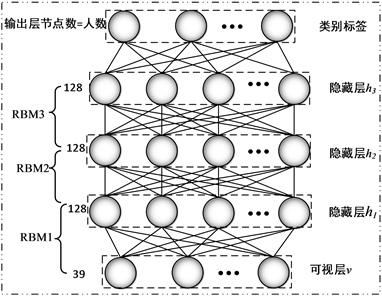# 基于深度学习的小样本声纹识别研究Research on Small Sample Voiceprint Recognition Based on Deep Learning

• 全文下载: PDF(814KB)    PP.30-37   DOI: 10.12677/AAM.2020.91004
• 下载量: 93  浏览量: 162

This paper studies the problem of small sample voiceprint recognition. In the experiment, the 39-dimensional features composed of the Mel cepstral coefficient and its dynamic differential coefficients are used as the basic acoustic features, and the basic acoustic features are extracted from the 128-dimensional depth acoustics through a deep belief network stacked by a three-layer restricted Boltzmann machine. Finally through the support vector machine and random forest for voiceprint recognition. Training deep belief networks, each speaker to choose short speech signal of small sample data as the network training set, trained deep belief network model at the same time as the depth of the acoustic feature extraction, with the characteristics of the extractor on the depth of the training focus on the speaker voice signal extraction acoustic characteristics, the generalization ability of the depth acoustic feature extractor is further verified. The experimental results show that the soundprint recognition model designed in this paper has high recognition accuracy, and the depth feature extractor has better generalization ability.

1. 引言

2. 深度学习方法概述

2.1. 基于深度置信网络的参数预训练

$E\left(v,h|\theta \right)=-\underset{i=1}{\overset{n}{\sum }}{\alpha }_{i}{v}_{i}-\underset{j=1}{\overset{m}{\sum }}{b}_{j}{h}_{j}-\underset{i=1}{\overset{n}{\sum }}\underset{j=1}{\overset{m}{\sum }}{v}_{i}{W}_{ij}{h}_{j}$

$P\left(v,h|\theta \right)=\frac{{\text{e}}^{-E\left(v,h|\theta \right)}}{Z\left(\theta \right)},\text{\hspace{0.17em}}Z\left(\theta \right)=\underset{v,h}{\sum }{\text{e}}^{-E\left(v,h|\theta \right)}$

$p\left({h}_{j}=1|v,\theta \right)=\sigma \left({b}_{j}+\underset{j}{\sum }{v}_{i}{W}_{ij}\right)$

$p\left({v}_{i}=1|h,\theta \right)=\sigma \left({a}_{i}+\underset{i}{\sum }{W}_{ij}{h}_{j}\right)$

RBM的优化目标是要最大化可见层节点概率分布，在训练过程中可以通过对比散度算法(contrastive divergence, CD)来得到模型中的参数。对比散度算法的输入是一个训练样本 ${x}_{0}$，设隐层单元个数m，学习率 $є$，最大训练周期T，输出是连接权重矩阵W，可见层偏置a，隐藏层偏置向量b，算法描述如下：

For $t=1,2,\cdots ,T$

For $j=1,2,\cdots ,m$ (对所有隐单元)；

End For

For $i=1,2,\cdots ,n$ (对所有可见单元)；

End For

For $j=1,2,\cdots ,m$ (对所有隐单元)；

End For

$\begin{array}{l}W←W+є\left(P\left({h}_{1}=1|{v}_{1}\right){v}_{1}^{\text{T}}-P\left({h}_{2}=1|{v}_{2}\right){v}_{2}^{\text{T}}\right)\\ a←a+є\left({v}_{1}-{v}_{2}\right)\\ b←b+є\left(P\left({h}_{1}=1|{v}_{1}\right)-P\left({h}_{2}=1|{v}_{2}\right)\right)\end{array}$

2.2. 基于反向传播算法的参数调优

${v}^{k+1}={\omega }^{k}{h}^{k}+{b}^{k}$ ${h}^{k}=\sigma \left({v}^{k}\right)$

${p}_{s}=\frac{\mathrm{exp}\left({v}_{s}^{N+1}\right)}{\underset{j}{\sum }\mathrm{exp}\left({v}_{s}^{N+1}\right)}$

$L=-\underset{s}{\sum }{d}_{s}\mathrm{log}{p}_{s}$

3. 有监督分类器

3.1. 支持向量机

$f\left(x\right)=\omega x+b$

$f\left(x\right)>0$，则待测样本分为一类；若 $f\left(x\right)<0$，待测样本分为另一类。

$\begin{array}{l}\text{Minimize}\Phi \left(\omega ,b\right)=\frac{1}{2}{‖\omega ‖}^{2}+C{\sum }_{i=1}^{n}{\xi }_{i}\\ \text{s}\text{.t}\text{.}{y}_{i}\left(\omega \cdot x+b\right)-1+{\xi }_{i}\ge 0,\text{}i=1,2,\cdots ,n\end{array}$

$f\left(x\right)=\mathrm{sgn}\left\{\left(w\cdot x\right)+b\right\}=\mathrm{sgn}\left\{\underset{i=1}{\overset{m}{\sum }}{\alpha }_{i}^{\ast }{y}_{i}\left({x}_{i}\cdot x\right)+{b}^{\ast }\right\}$

$f\left(x\right)=\text{sign}\left(\underset{i=1}{\overset{n}{\sum }}{\alpha }_{i}^{*}{y}_{i}K\left({x}_{i}\cdot x\right)+{y}_{j}-\underset{i=1}{\overset{n}{\sum }}{\alpha }_{i}^{*}{y}_{i}K\left({x}_{i}\cdot {x}_{j}\right)\right)$

3.2. 随机森林

Step 1：对于 $t=1,2,\cdots ,T$

1) 对训练数据集D采用自助法采样，得到包含m个样本的采样集 ${D}_{t}$

2) 使用采样集 ${D}_{t}$ 训练第t个决策树模型 ${G}_{t}\left(x\right)$，从所有特征中随机抽样出一个包含k个特征的子集，使用CART算法构建决策树。

Step 2：若为分类问题，则使用T个模型的类别标签进行投票预测；若为回归问题，则用简单平均法进行预测。

4. 实验设置与结果分析

4.1. 声学特征提取

4.1.1. 实验数据设置及MFCC提取

4.1.2. 深度声学特征d-vector

4.2. 基于深度学习的声纹识别结果Table 1. Experimental data set settingsFigure 1. DBN structureTable 2. Recognition rate of MFCC (39 dim) in the modelTable 3. Recognition rate of d-vector (128 dim) in the model

4.3. 深度神经网络模型泛化验证Table 4. Model generalization capability verification results

5. 结论

NOTES

*通讯作者。

  Atal, B.S. (1976) Automatic Recognition of Speakers from Their Voices. Proceedings of the IEEE, 64, 460-475. https://doi.org/10.1109/PROC.1976.10155  Hermansky, H. (1990) Perceptual Linear Predictive (PLP) Analysis of Speech. The Journal of the Acoustical Society of America, 87, 1738-1752. https://doi.org/10.1121/1.399423  Davis, S.B. (1980) Comparison of Parametric Representations for Monosyllabic Word Recognition in Continuously Spoken Sentences. IEEE Transactions on Acoustics, Speech, and Signal Processing, 28, 65-74. https://doi.org/10.1109/TASSP.1980.1163420  Furui, S. (1981) Cepstral Analysis Technique for Automatic Speaker Verification. IEEE Transactions on Acoustics Speech and Signal Processing, 29, 254-272. https://doi.org/10.1109/TASSP.1981.1163530  Burton, D.K. (1987) Text-Dependent Speaker Verification Using Vector Quantization Source Coding. IEEE Transactions on Acoustics Speech and Signal Processing, 35, 133-143. https://doi.org/10.1109/TASSP.1987.1165110  Soong, F.K. and Rosenberg, A.E. (1988) On the Use of Instantaneous and Transitional Spectral Information in Speaker Recognition. IEEE Transactions on Acoustics Speech & Signal Processing, 36, 871-879. https://doi.org/10.1109/29.1598  Naik, J.M., Netsch, L.P. and Doddington, G.R. (1989) Speaker Verification over Long Distance Telephone Lines. International Conference on Acoustics, Speech, and Signal Processing, Glasgow, 23-26 May 1989, 524-527.  Yang, Y., Ren, W., Hui, Z., et al. (2012) The Research of Voiceprint Recognition Based on Genetic Optimized RBF Neural Networks. IEEE International Conference on Computer Science & Automation Engineering, Zhangjiajie, 25-27 May 2012, 425-428. https://doi.org/10.1109/CSAE.2012.6272630  Abu, M.A., Zakariya, Q., et al. (2018) New Transformed Features Generated by Deep Bottleneck Extractor and a GMM-UBM Classifier for Speaker Age and Gender Classification. Neural Computing & Applications, 30, 2581-2593. https://doi.org/10.1007/s00521-017-2848-4  Variani, E., Lei, X., McDermott, E., Lopez Moreno, I. and Gonzalez-Dominguez, J. (2014) Deep Neural Networks for Small Footprint Text-Dependent Speaker Verification. IEEE International Conference on Acoustics, Speech and Signal Processing, Florence, 4-9 May 2014, 4052-4056. https://doi.org/10.1109/ICASSP.2014.6854363  Hinton, G., Deng, L., Yu, D., et al. (2012) Deep Neural Networks for Acoustic Modeling in Speech Recognition: The Shared Views of Four Research Groups. IEEE Signal Processing Magazine, 29, 82-97. https://doi.org/10.1109/MSP.2012.2205597  田垚, 蔡猛, 何亮, 刘加. 基于深度神经网络和Bottleneck特征的说话人识别系统[J]. 清华大学学报(自然科学版), 2016, 56(11): 1143-1148.  闫河, 董莺艳, 王鹏, 罗成, 李焕. 基于CNN-LSTM网络的声纹识别研究[J]. 计算机应用与软件, 2019, 36(4): 166-170.  Snyder, D., et al. (2017) Deep Neural Network-Based Speaker Embeddings for End-to-End Speaker Verification. Spoken Language Technology Workshop, San Diego, 13-16 December 2016, 165-170.  张春霞, 姬楠楠, 王冠伟. 受限玻尔兹曼机[J]. 工程数学学报, 2015, 32(2): 161-175.  Hearst, M.A. (1998) Support Vector Machines. IEEE Intelligent Systems & Their Applications, 13, 18-28. https://doi.org/10.1109/5254.708428  Ho, T.K. (1995) Random Decision Forests. International Conference on Document Analysis & Recognition, Montreal, 14-16 August 1995, 278-282.